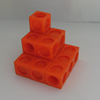#### You may also like### Picture Story

Can you see how this picture illustrates the formula for the sum of the first six cube numbers?### The Root of the Problem

Find the sum of this series of surds.### Sums of Powers - A Festive Story

A story for students about adding powers of integers - with a festive twist.

# Slick Summing

##### Age 14 to 16Challenge Level

Thanks to Lasse (from St. James Senior Boys' School), Fred (from Wembley High Technology College), Nishil (from Cambridge School), Rishi (from St. Matthew's Primary School), Zachary (from Sonoran Science Academy), Kaushal (from Overseas Family School), Rukkshana and Annie (from Fern Avenue Public School), Julia and Grace (from Inter-Lakes) and Julian (from British School Manila) for all the great answers. Here is just one of them, by Zachary:

First, let's work out a solution to 1+2+3+...+10.

When we pair the first and last term, 2nd and 9th term, 3rd and 8th term, ..., we get sums of 11. We can list the pairs:

• 1 and 10
• 2 and 9
• 3 and 8
• 4 and 7
• 5 and 6

There are 5 pairs of sums that add up to 11, and so we get a sum of 5*11 = 55.

Now, we need to find 1+2+3+...+20. Now listing out the terms would be a bad idea, so let's think about how many terms that was in the sum of 1-10. There were 5 pairs in 1-10. So, if the same rules apply, then there would be 10 pairs in the sum of 1-20. Now, we find the sum by adding the first and the last terms of the sequence. 1+20= 21. We have 10 pairs of 21, and the sum is 10*21= 210.

We will do the same thing for 1-100. 100/2= 50 pairs. 1+100= 101. 50 pairs of 101 is 50*101= 5050.

Now, we have the sum of 40-100. This is harder to solve, because the first term isn't 1. So, let's start by finding the number of terms. First, we must realize that in the set of numbers from n to m, there are m-n+1 terms. So here we have the numbers from 40 to 100, so there are 61 terms. We use the same method, even though there are not an even number of terms. 61/2=30.5. 100+40= 140. 140*30.5= 4270.

Brilliant! Lasse pointed out that sums like this are called 'arithmetic series' - well spotted. Kaushal proved a general formula for us:

The sum 1 + 2 + 3 + 4 ... + n-1 + n can be rearranged:

(1 + n) + (2 + (n - 1)) + (3 + (n - 2)) +...

where each term is in the form (x + (n - (x-1)). This simplifies to:

(1 + n) + (1 + n) + (1 + n) +...

The number of ''(1 + n)''s that are added is n/2 since (x + n - (x-1)) is always n + 1. Therefore,

$1 + 2 + 3 + 4 +\dots + n-1 + n = \frac{n^2 + n}{2}$.

Julian gave us the following answers:

• 1+3+5+...+17+19 is (20*10)/2 which is 100
• 2+4+6+...+18+20 is (22*10)/2 which is 110
• 42+44+46...+98+100 is (142*30)/2 which is 2130

Great - but where did these come from? (Can anyone else work it out?)